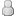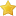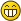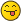Cure Inc?
01-09-2014, 08:17 PM (This post was last modified: 03-09-2014 12:10 AM by joemeng967.)
Post: #1
 joemeng967Junior MemberPosts: 7 Joined: May 2014
Cure Inc?
I have created a Small Sample Coding in PHP (More to Come) of "Cure Inc." a possible addition to plague inc where you work as the person who tries to cure it.
If there is someone who could help possibly translate it into C++ it would be appreciated.
I don't know any complex Formulas to simulate disease progression or spread, so if anyone could help me out with that it would be appreciated.
Code:
```<?php \$population = array("China" => "1400000000", "India" => "1300000000", "Africa" => "1000000000", "Middle East" => "600000000", "Europe" => "700000000", "Russia" => "170000000", "Japan" => "127000000", "Central Asia" => "67000000", "North America" => "565000000", "South America" => "386000000"); \$world = array_sum(\$population); \$infected = array("China" => "0", "India" => "0", "Africa" => "0", "Middle East" => "0", "Europe" => "0", "Russia" => "0", "Japan" => "0", "Central Asia" => "0", "North America" => "0", "South America" => "0"); ?>```
I Honestly Don't know much php, there is alot more but i can't get it to work, so any help would be appreciated!
It would be cool if eventually it could be merged with the main game.
Necroa Virus Equivalent
Its Supposed to Use Javascript along with PHP to simulate the Game Session, trying to convert to C++
Code:
```include “array.php”; include “mysql_query.php”; Function GetNumberOfInfected(\$infect){ switch(\$infect){ case 1: \$numbe1 = mt_rand(1, 1000); \$numbe2 = mt_rand(1, 1000); if(\$numbe1 == \$numbe2){ \$infect1 = mt_rand(0, 10); return \$infect1; break; }else{ return 0; break; } case 2: \$numbe3 = mt_rand(1, 250); \$numbe4 = mt_rand(1, 250); if(\$numbe3 == \$numbe4){ \$infect2 = mt_rand(5, 20); return \$infect2; break; }else{ return 0; break; } case 3: \$numbe5 = mt_rand(1, 125); \$numbe6 = mt_rand(1, 125); if(\$numbe5 == \$numbe6){ \$infect3 = mt_rand(20, 80); return \$infect3; break; }else{ return 0; break; } case 4: \$numbe7 = mt_rand(1, 50); \$numbe8 = mt_rand(1, 50); if(\$numbe7 == \$numbe8){ \$infect4 = mt_rand(80, 320); return \$infect4; break; }else{ \$r = mt_rand(50, 250); return \$r; break; } case 5: \$numbe9 = mt_rand(1, 10); \$numbe10 = mt_rand(1, 10); if(\$numbe9 == \$numbe10){ \$infect5 = mt_rand(320, 1280); return \$infect5; break; }else{ \$rr = mt_rand(100, 300); return \$rr; break; } case 6: \$numbe11 = mt_rand(1, 5); \$numbe12 = mt_rand(1, 5); if(\$numbe11 == \$numbe12){ \$infect6 = mt_rand(1280, 5120); return \$infect6; }else{ \$rrr = mt_rand(200, 600); return \$rrr; }}} Function GetNumberOfDead(\$lethality){ switch(\$lethality){ case 1: \$number1 = mt_rand(1, 1000); \$number2 = mt_rand(1, 1000); if(\$number1 == \$number2){ \$dead1 = mt_rand(0, 10); return \$dead1; break; }else{ return 0; break; } case 2: \$number3 = mt_rand(1, 250); \$number4 = mt_rand(1, 250); if(\$number3 == \$number4){ \$dead2 = mt_rand(5, 50); return \$dead2; break; }else{ return 0; break; } case 3: \$number5 = mt_rand(1, 125); \$number6 = mt_rand(1, 125); if(\$number5 == \$number6){ \$dead3 = mt_rand(50, 100); return \$dead3; break; }else{ return 0; break; } case 4: \$number7 = mt_rand(1, 50); \$number8 = mt_rand(1, 50); if(\$number7 == \$number8){ \$dead4 = mt_rand(100, 1000); return \$dead4; break; }else{ \$d = mt_rand(50, 250); return \$d; break; } case 5: \$number9 = mt_rand(1, 10); \$number10 = mt_rand(1, 10); if(\$number9 == \$number10){ \$dead5 = mt_rand(1000, 10000); return \$dead5; break; }else{ \$dd = mt_rand(100, 300); return \$dd; break; } case 6: \$number11 = mt_rand(1, 5); \$number12 = mt_rand(1, 5); if(\$number11 == \$number12){ \$dead6 = mt_rand(2000, 15000); return \$dead6; }else{ \$ddd = mt_rand(200, 600); return \$ddd; }}} Function GetNumberOfZombified(\$combat){ switch(\$combat){ case 1: \$numb1 = mt_rand(1, 1000); \$numb2 = mt_rand(1, 1000); if(\$numb1 == \$numb2){ \$zom1 = mt_rand(0, 1000); return \$zom1; break; }else{ \$t = mt_rand(0, 300); return \$t; break; } case 2: \$numb3 = mt_rand(1, 250); \$numb4 = mt_rand(1, 250); if(\$numb3 == \$numb4){ \$zom2 = mt_rand(500, 2000); return \$zom2; break; }else{ \$tt = mt_rand(100, 600); return \$tt; break; } case 3: \$numb5 = mt_rand(1, 125); \$numb6 = mt_rand(1, 125); if(\$numb5 == \$numb6){ \$zom3 = mt_rand(2000, 8000); return \$zom3; break; }else{ \$ttt = mt_rand(300, 900); return \$ttt; break; } case 4: \$numb7 = mt_rand(1, 50); \$numb8 = mt_rand(1, 50); if(\$numb7 == \$numb8){ \$zom4 = mt_rand(6000, 27000); return \$zom4; break; }else{ \$tttt = mt_rand(900, 2700); return \$tttt; break; } case 5: \$numb9 = mt_rand(1, 10); \$numb10 = mt_rand(1, 10); if(\$numb9 == \$numb10){ \$zom5 = mt_rand(18000, 72000); return \$zom5; break; }else{ \$ttttt = mt_rand(2700, 7200); return \$ttttt; break; } case 6: \$numb11 = mt_rand(1, 5); \$numb12 = mt_rand(1, 5); if(\$numb11 == \$numb12){ \$zom6 = mt_rand(20000, 80000); return \$zom6; }else{ \$tttttt = mt_rand(7200, 54000); return \$ttttt; }}} Function ZombiesKilled(\$combath){ switch(\$combath){ case 1: \$num1 = mt_rand(1, 1000); \$num2 = mt_rand(1, 1000); if(\$num1 == \$num2){ \$zk1 = mt_rand(0, 500); return \$zk1; break; }else{ return 0; break; } case 2: \$num3 = mt_rand(1, 250); \$num4 = mt_rand(1, 250); if(\$num3 == \$num4){ \$zk2 = mt_rand(250, 1000); return \$zk2; break; }else{ return 0; break; } case 3: \$numb5 = mt_rand(1, 125); \$numb6 = mt_rand(1, 125); if(\$numb5 == \$numb6){ \$zk3 = mt_rand(1000, 4000); return \$zk3; break; }else{ \$y = mt_rand(150, 450); return \$y; break; } case 4: \$numb7 = mt_rand(1, 50); \$numb8 = mt_rand(1, 50); if(\$numb7 == \$numb8){ \$zk4 = mt_rand(18000, 54000); return \$zk4; break; }else{ \$yy = mt_rand(1000, 3000); return \$yy; break; } case 5: \$numb9 = mt_rand(1, 10); \$numb10 = mt_rand(1, 10); if(\$numb9 == \$numb10){ \$zk5 = mt_rand(36000, 180000); return \$zk5; break; }else{ \$yyy = mt_rand(3000, 7500); return \$yyy; break; } case 6: \$numb11 = mt_rand(1, 5); \$numb12 = mt_rand(1, 5); if(\$numb11 == \$numb12){ \$zom6 = mt_rand(40000, 160000); return \$zom6; }else{ \$yyyy = mt_rand(7500, 60000); return \$yyyy; }}} Function ResearchCure(\$amount){ \$nu = mt_rand(1, 5); \$nu1 = mt_rand(1, 5); if(\$nu == \$nu1){ \$mysqli->query(“UPDATE gamestats SET cure = cure + 1”); } } Function DelayCure(){ \$mysqli->query(“UPDATE gamestats SET cure = cure - 5”); } Function DeployCure(){ if(\$_SESSION[‘cure’] = 100){ session_unset(); http_get(“zombies.html?lose=1”); } Function Dead(\$id, \$amount){ \$_SESSION[‘worldpopulation’] = \$_SESSION[‘worldpopulation’] - \$amount; \$_SESSION[‘alive’][\$id] = \$_SESSION[‘alive’][\$id] - \$amount; \$_SESSION[‘dead’][\$id] = \$_SESSION[‘dead’][\$id] + \$amount; \$_SESSION[‘worlddead’] = \$_SESSION[‘worlddead’] + \$amount; } Function CureResearch(\$amount){ ResearchCure(\$amount); } Function FirstInfect(\$id){ \$_SESSION[‘infected’][\$id] = 1; \$_SESSION[‘healthy’][\$id] = \$_SESSION[‘country’][\$id] - 1; \$_SESSION[‘worldinfected’] = 1; \$_SESSION[‘worldhealthy’] = \$_SESSION[‘worldpopulation’] - 1; } Function InfectNew(\$amount, \$id){ \$_SESSION[‘infected’][\$id] = \$_SESSION[‘infected’][\$id] + \$amount; \$_SESSION[‘healthy’][\$id] = \$_SESSION[‘healthy’][\$id] - \$amount; \$_SESSION[‘worldinfected’] = \$_SESSION[‘worldinfected’] + \$amount; \$_SESSION[‘worldhealthy’] = \$_SESSION[‘worldhealthy’] - \$amount; } Function InfectNewCountry(\$infectionchance){ switch(\$infectionchance){ case 1: \$n = mt_rand(1, 1000); \$n1 = mt_rand(1, 1000); if(\$n == \$n1){ \$newcountry = array_rand(\$country, 1); FirstInfect(\$newcountry); } case 2: \$n2 = mt_rand(1, 100); \$n3 = mt_rand(1, 100); if(\$n2 == \$n3){ \$newcountry2 = array_rand(\$country, 1); FirstInfect(\$newcountry2); } case 3: \$n4 = mt_rand(1, 10); \$n5 = mt_rand(1, 10); if(\$n4 == \$n5){ \$newcountry3 = array_rand(\$country, 1); FirstInfect(\$newcountry3); } case 4: \$n6 = mt_rand(1, 5); \$n7 = mt_rand(1, 5); if(\$n6 == \$n7){ \$newcountry4 = array_rand(\$country, 1); FirstInfect(\$newcountry4); }} \$firstcountry = \$_GET[‘country’]; \$symptom = \$_GET[‘symptom’]; \$infectivity = array_sum(\$infectivity); \$lethalness = array_sum(\$symptoms); \$command = \$_GET[‘command’]; \$infectedcountries = \$firstcountry; if(\$infectedcountries == \$_SESSION[‘country’][‘Middle East’]){ http_get(“zombies.html?newsfeed=civilwar”); } FirstInfect(\$firstcountry); while(\$command == 1){ \$infection = GetNumberOfInfected(\$infectivity); \$deadguys = GetNumberOfDead(\$lethalness); InfectNew(\$infectivity, \$firstcountry); Dead(\$firstcountry, \$deadguys); CureResearch(\$firstcountry); InfectNewCountry(\$Infectivity); if(\$deadguys == \$_SESSION[‘worldpopulation’]){ session_unset(): http_get(“zombies.html?lose=2”); }```
"Vampire Plague Disease Progression Code Handling"
Code:
```<?php if(\$severity > 4 && < 6){ return "secondstage"; }else{ if(\$severity > 6 && < 8){ return "thirdstage"; }else{ if(\$severity > 8 && < 10){ return "fourthstage"; }}```
07-09-2014, 07:42 PM
Post: #2ManganManAspie owned by Neurax WormPosts: 112 Joined: May 2014
RE: Cure Inc?
Looks interesting.
This feat should be used in PvP matchesSpeaking fluently in Polish and in basic English.
Oops Combo in reality« Next Oldest | Next Newest »

Forum Jump:

User(s) browsing this thread: 1 Guest(s)